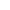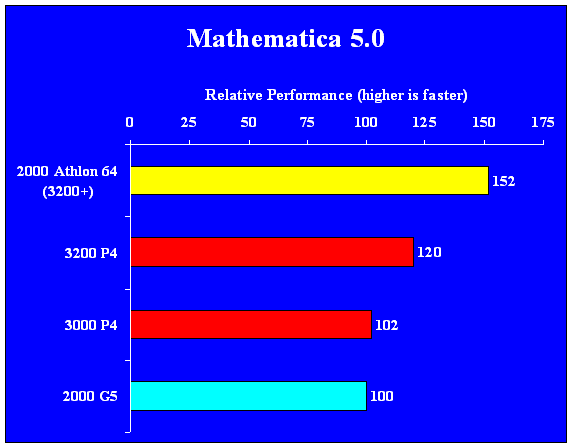Mathematica 5.0Mathematica 5.0 is a mathematics modelling application.Scores are relative performance based on a geometric mean of normalized (on 2000 MHz G5) scores from 15 subtests using the MMA 5.0 benchmark.  To generate the normalized subtest scores, the inverse of the subtest time for a given processor was divided by the inverse of subtest time for the base processor (2000 G5) and then multiplied by 100% (inverse used because lower times equal faster performance); this was done separately for each subtest.  To generate the overall score for a given processor, the geometric mean was calculated based on all of the individual subtest normalized scores for that processor.  This means that each subtest was equally weighted regardless of how long it took to run relative to other subtests.  This benchmark only tests single-processor performance.The P4 and Athlon 64 scores are based on the Windows version.  The version for the Athlon 64 / Opteron running 64-bit Linux is significantly faster than the Windows version.For a point of reference; the fastest clockspeeds available in OEM systems as of 21 May 2004 are: 2000 MHz G5, 2200 MHz Athlon 64, 2400 MHz Opteron, 3400 MHz P4, and 3200 MHz Xeon. Links: HOME MMA 5.0 benchmark subtest timesMMA 5.0 benchmark Last Updated: May 21, 2004